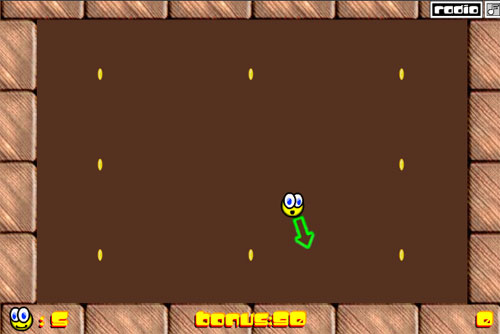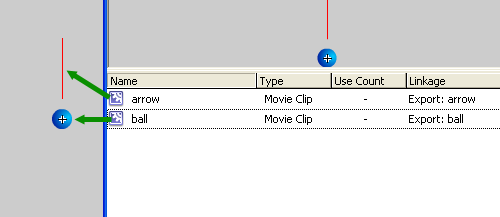# A strange way to move the player with Flash

When I look for some inspiration I browse some sites where talented designers post.

One of them is Newgrounds, almost all famous games are listed in it. I found a pretty good game called Pacco with a strange way to move the player. I have to be honest, I did not like the game itself but I liked something about the way you move the player.I guess I can do a quite nice game starting from it.

First of all, play a bit Pacco, the follow the tutorial.

The character

The main character is a ball with a rotating arrow. Here it is how I did it: I created a movieclip linkaged as ball and inside of it I put the movieclip linkaged as arrowThe picture represents the ball movieclip, not the scene

The arrow must rotate, no matter if clockwise or counter-clockwise at the moment

```attachMovie("ball", "ball", 1, {_x:300, _y:200});
ball.onEnterFrame = function() {
this.arrow._rotation += 5;
};```

Line 1: crete the ball (with the arrow inside of it)

Lines 2-4: rotates the arrow of 5 degrees clockwisely at every frame

Press the mouse to stop rotation

This does not happen in Pacco but I am going to change a bit the gameplay. I want the arrow to stop when I press the mouse button

```rotate = true;
attachMovie("ball", "ball", 1, {_x:300, _y:200});
ball.onEnterFrame = function() {
if (rotate) {
this.arrow._rotation += 5;
}
};
function onMouseDown() {
rotate = false;
}```

Line 1: Boolean variable indicating that the arrow must rotate

Line 4: If rotate is true (and it’s true by default) then proceed with the rotation routine

Lines 8-10: If the mouse button is pressed (I mean pressed, not released) the rotate variable turns to false

Now the arrow stops rotating when we press the mouse button

Charging the power

The next step is to chage the power when we hold the mouse button pressed.

I edited the ball movieclip inserting a dynamic text field called pwr… just to show the power while it charges

```rotate = true;
power = 0;
attachMovie("ball", "ball", 1, {_x:300, _y:200});
ball.onEnterFrame = function() {
if (rotate) {
this.arrow._rotation += 5;
} else {
power++;
if (power>50) {
power = 50;
}
}
this.pwr.text = power;
};
function onMouseDown() {
rotate = false;
}```

Line 2: Initializing the power to 0

Lines 7-12: If the arrow is not rotating (this means the player pressed the mouse) then start charging the power. Lines 9-11 prevents the power to raise over 50

Line 13: Displays the power writing its value in the text field

Now we have a charging power but you can notice that the power charges even if we stop holding the mouse down and there is no way to reset it.

Charging the power – step 2

Now I want the power to reset if I release the mouse button, and want the charged power to spread along arrow direction

```rotate = true;
power = 0;
dir = 1;
ballspeed_x = 0;
ballspeed_y = 0;
attachMovie("ball", "ball", 1, {_x:300, _y:200});
ball.onEnterFrame = function() {
if (rotate) {
this.arrow._rotation += 5*dir;
} else {
power++;
if (power>50) {
power = 50;
}
}
this.pwr.text = power;
};
function onMouseDown() {
rotate = false;
}
function onMouseUp() {
if (!rotate) {
rotate = true;
ballspeed_x += Math.sin(ball.arrow._rotation*Math.PI/180)*power/10;
ballspeed_y += Math.cos(ball.arrow._rotation*Math.PI/180)*power/10;
power = 0;
dir *= -1;
}
}```

Lines 4-5: Initialize ball speed at zero.

Lines 21-29: Function to call when the mouse button is released, only if rotate value is false.

Line 23: Rotate value is set to true again

Lines 24-25: ball speed x and y are calculated according to rotation and power using trigonometry as explained in this tutorial

Line 26: resets the power

Line 27: Changes the rotation way. If you noticed lines 3 and 9 you can understand how dir value affects the way of rotation. I want the rotation to change every time I release the mouse.

Now it’s time to move the player

Moving the player

To have the ball moving we need this actionscript

```rotate = true;
power = 0;
dir = 1;
ballspeed_x = 0;
ballspeed_y = 0;
friction = 0.99;
attachMovie("ball", "ball", 1, {_x:300, _y:200});
ball.onEnterFrame = function() {
if (rotate) {
this.arrow._rotation += 5*dir;
} else {
power++;
if (power>50) {
power = 50;
}
}
this._x += ballspeed_x;
this._y -= ballspeed_y;
ballspeed_x *= friction;
ballspeed_y *= friction;
this.pwr.text = power;
};
function onMouseDown() {
rotate = false;
}
function onMouseUp() {
if (!rotate) {
rotate = true;
ballspeed_x += Math.sin(ball.arrow._rotation*Math.PI/180)*power/10;
ballspeed_y += Math.cos(ball.arrow._rotation*Math.PI/180)*power/10;
power = 0;
dir *= -1;
}
}```

Line 6: Defining the friction as we do not want the player to move forever. You can find a tutorial about friction here

Lines 17-18: Updating ball _x and _y according to ballspeed_x and ballspeed_y values

Lines 19-20: Applying the friction to ballspeed_x and ballspeed_y

Finally we have the moving ball!

Time to introduce some obstacles

The wall

The wall is the king of all obstacles. Obey the wall! I do it everyday.

I created a new movieclip linkaged as wall and changed the actionscript:

```rotate = true;
power = 0;
dir = 1;
ballspeed_x = 0;
ballspeed_y = 0;
friction = 0.99;
attachMovie("ball", "ball", 1, {_x:300, _y:200});
attachMovie("wall", "wall", 2);
ball.onEnterFrame = function() {
if (rotate) {
this.arrow._rotation += 5*dir;
} else {
power++;
if (power>50) {
power = 50;
}
}
this._x += ballspeed_x;
this._y -= ballspeed_y;
ballspeed_x *= friction;
ballspeed_y *= friction;
this.pwr.text = power;
if (wall.hitTest(this._x, this._y-10, true) or wall.hitTest(this._x, this._y+10, true)) {
ballspeed_y *= -1;
}
if (wall.hitTest(this._x+10, this._y, true) or wall.hitTest(this._x-10, this._y, true)) {
ballspeed_x *= -1;
}
};
function onMouseDown() {
rotate = false;
}
function onMouseUp() {
if (!rotate) {
rotate = true;
ballspeed_x += Math.sin(ball.arrow._rotation*Math.PI/180)*power/10;
ballspeed_y += Math.cos(ball.arrow._rotation*Math.PI/180)*power/10;
power = 0;
dir *= -1;
}
}```

Line 8: Attaching the wall in the scene

Lines 23-25: if the upper or lower part of the ball collides with the wall, I invert ball’s y speed because I am sure I touched the “ceiling” or the “floor”. For more information about hitTest method refer to this tutorial

Lines 26-28: Same thing with the leftmost and the rightmost part of the ball, inverting the x speed.

Caution: use this method only if you are SURE you have only horizontal or vertical straight walls. If you plan to put obstacles of different shapes or rotations, then you should apply the bounce method explained in this tutorial.

I am realizing I wrote a lot of tutorials… everytime I try to introduce something new I end writing “as said in this tutorial”. This should reward regular readers increasing their knowledge without studying all tuts in a time.

Anyway, that’s the game “finished”. It’s not over of course, as I plan to develop some new ideas starting from this tutorial.

214 GAME PROTOTYPES EXPLAINED WITH SOURCE CODE
// 1+2=3
// 10000000
// 2 Cars
// 2048
// Avoider
// Ballz
// Block it
// Blockage
// Bloons
// Boids
// Bombuzal
// Breakout
// Bricks
// Columns
// CubesOut
// Dots
// DROP'd
// Dudeski
// Eskiv
// Filler
// Fling
// Globe
// HookPod
// Hundreds
// InkTd
// Iromeku
// Lumines
// Magick
// MagOrMin
// Maze
// Memdot
// Nano War
// Nodes
// o:anquan
// Ononmin
// Pacco
// Phyballs
// Platform
// Poker
// Pool
// Poux
// Pudi
// qomp
// Racing
// Renju
// SameGame
// Security
// Sling
// Slingy
// Sokoban
// Splitter
// Sproing
// Stack
// Stringy
// Sudoku
// Tetris
// Threes
// Toony
// Turn
// TwinSpin
// vvvvvv
// Wordle
// Worms
// Yanga
// Zhed
// zNumbers